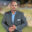Trusted answers to developer questions
Trusted Answers to Developer Questions

Related Tags

machine learning
loss
communitycreator

# What is mean square error in machine learning?Kedar Kodgire

Grokking Modern System Design Interview for Engineers & Managers

Ace your System Design Interview and take your career to the next level. Learn to handle the design of applications like Netflix, Quora, Facebook, Uber, and many more in a 45-min interview. Learn the RESHADED framework for architecting web-scale applications by determining requirements, constraints, and assumptions before diving into a step-by-step design process.

The machine learning model provides likely outcomes of a question based on historical data. Using loss functions, we can measure how far a predicted value is from its actual value. Mean square error is one of the commonly used loss functions.

### Measuring loss

To understand this theory, let’s consider a plane with an X and Y-axis.

### Explanation

In the diagram above:

1. The red dots indicate the data points.
2. The green line represents the line that we fitted on data points.
3. The blue dot indicates the predicted value.
4. The purple line suggests the loss.

As we can see, the predicted value is away from the actual value, and is represented by x8 and y8’. This indicates we have a loss, and it is calculated by y8-y8’. This provides us the difference between the predicted and actual values at x8. We can do this for all of the data points.

Let’s consider another example with the X and Y-axis and two data points.

### Explanation

In the diagram above:

1. Yellow dots represent data points.
2. The green line is a correctly fitted model.
3. The red line is a fallacious model.

To calculate the error, we perform the following mathematical operations.

• y1 - y1’ = positive value as y1 > y1’
• y2 - y2’ = negative value as y2 < y2’

We need to add them to calculate the total loss, i.e., (y1-y1’) + (y2-y2’) ~ 0. From the diagram, we can see that the red line is equally away from both points. So when we add them, we get answers of approximately zero. This is incorrect because we have a fair amount of loss in our function.

### Mean square error

To avoid this scenario, we square the values:

$(y1-y1')^{2}$ + $(y2-y2')^{2}$ >> 0

Now we see that loss is greater than zero. This is because the purple line doesn’t fit the points well. Hence, the loss should be greater than zero.

Now we take the mean of the results as we divide by the number of points.

$\frac{1}{2}(y1-y1')^{2}$ + $(y2-y2')^{2}$ >> 0

The generic equation looks like the following:

$\frac{1}{n}\sum(y1-y1')^{2}$

This is exactly why the loss function is called mean square error.

RELATED TAGS

machine learning
loss
communitycreator

CONTRIBUTORKedar Kodgire

Grokking Modern System Design Interview for Engineers & Managers

Ace your System Design Interview and take your career to the next level. Learn to handle the design of applications like Netflix, Quora, Facebook, Uber, and many more in a 45-min interview. Learn the RESHADED framework for architecting web-scale applications by determining requirements, constraints, and assumptions before diving into a step-by-step design process.

Keep Exploring

Learn in-demand tech skills in half the time